算法到图像处理算法_图像处理算法 和视频处理算法的区别 - CSDN
• 一些我学习数字图像处理中的心得和建议，欢迎有兴趣的朋友共同参与讨论。工欲善其事，必先利其器。有时一些方法论之类的东西往往能让你少走很多弯路
陆放翁诗云：“纸上得来终觉浅，绝知此事要躬行”，此乃亘古不变之真理也。图像处理研究的一个很大特点就在于，它是一种和实际应用紧密联系的实用型学科。所有的算法都是针对实际中遇到的问题而存在的。无论是我们新提出了一个算法，还是我们新学习了一个算法，都可以直接写一个程序来检验它的处理效果。而且这种“效果”往往还是一目了然的。因为图像处理的输入和输出都是图像，图像就是用来给人看的。这种直观性是其它研究领域所很难具备的。例如，当年何恺明博士向CVPR提交其关于图像去雾算法的论文时，审稿人就很惊讶于这么简单的方法居然有这么意外的效果。于是其中就有好奇的审稿人直接动手编程实现了他的算法。正所谓，是骡子是马，拉出来溜溜。他算法的效果最终征服了审稿人，也征服了大会的评委会，最终那届的最佳论文奖就首次颁给了来自亚洲的研究者。而之于正在学习或研究图像算法的同学而言，动手实践的意义就更大了。不要以为你听老师或别人讲了一些算法，或者自己看看冈萨雷斯书上的描述，就觉得自己好像已经get了什么。除非你真的自己能把程序写出了，否则其实你什么都没懂，最多也就是似懂非懂。这一点我最有发言权。很多算法我感觉我也学得不错，但其实我在写Magic House的时候仍然花了很多时间去调试，因为在写的过程中，其实问题是层出不穷的。例如，我在写图像傅立叶逆变换的时候，我感觉这个算法我已经理解的很到位了，但是做逆变换的时候就是得不到原图，而是一篇黑。问题到底出在哪里我其实断断续续想了挺长时间。有一天突然开窍了，因为傅立叶变换的结果存在文件里，其实只是保存了实数的部分，虚数部分根本就没存。因为你在屏幕上看傅立叶变换结果图的时候，看到的也只是实数部分。尽管你可能遇到的问题跟我不一样，但遇到这样或那样的问题总是肯定的。我总结了自己锻炼编写图像处理代码的几个原因：1）一方面你会发现过去你的理解可能是有偏差的，因为按照你那个想法去实现的代码效果可能并不理想，那问题出在哪里就很值得你思索；2）另一方面，你自己在实现的过程中很可能发现原作者算法的不足（比如我在实现暗通道算法时就发现这个算法处理大面积的天空时会有问题），然后你进一步研究的方向就来了！这在我看来似乎是发paper最容易的方法。3）我不得不告诉你，很多paper是会骗人的！中国有学术腐败，国外也有学术造假。洛必达把他老师伯努利教给他的方法写进了自己的书里，于是便有了今天你所学到的洛必达法则。从这个角度来看，18世纪的欧洲就已经有学术腐败了。有些作者paper里的效果很可能是PS过的，所以你按照他的方法，无论怎么做也不可能得到他的效果，这一点如果你不试试，就很可能被骗。4）写代码本身就是一项本领或者基本功。你将来还很有可能要靠他生存。曲不离口、拳不离手。你现在不好好练，将来很可能作茧自缚。说到这里我就不禁要提一句。懒惰既是推动人类创新的两大动力之一，但也可能是害得你“啥都不会，只会坐等靠要”、最终一事无成的毒草。就像中国过去搞扶贫只会一味的输血，本来还有二亩薄田可以耕种，现在政府有救济款，索性连田也懒得种了，结果导致某些地方越扶越贫。现在很多人在网上一张嘴就是“有没有源代码下载”？或者买书看书也是挑那种“带光盘的”或者满篇满篇就只有代码的。抄来的过程当然轻松，但是知识、技术学会了是自己的，抄来的东西始终是别人的。不是说网上的代码、资源不可以参考，但是你绝对不应该对此产生依赖心理，人还是应该靠自己。如果你写不出来，只能说明你还没真正学会，学懂。何况真正有技术含量的东西，你永远也抄不来。两弹一星我们也曾想让苏联帮忙，但人家凭啥给你？结果怎么样，还不得靠中国人自己吗？要知道，这个技术积累的过程本身也很重要。说道实践编程，你要么用C/C++，要么用Matlab，可能还有其他小众的（比如我有时也用R做图像处理），但大体上总逃不过这两大阵营。有人说他用Java或者Python， Anyway这其实并不重要。我的讨论还是以C/C++和Matlab为主体来讲。Matlab当然比较好用，什么卷积、直方图、高斯滤波，一个函数搞定！C/C++如果结合OpenCV基本可以达到同样的效果。当这绝对是后话。如果你是初学，最好能踏踏实实一点一滴把最基本的图像处理算法实现一下。千里之行，始于足下。九层之台，起于累土。因为建立一个完整的体系思维和拥有一个扎实的基础特别重要。一些看似高大上的算法都是在这些不起眼的算法的堆砌组合下实现的。比如SIFT特征构建时，你会遇到直方图、高斯滤波和图像金字塔这些非常基础的原件。当你看到这些东西的时候，你就应该有马上觉悟到它们的各种特性和作用的能力。这对于你理解SIFT很有帮助。如果你具备了这种素质，详细我，你再在matlab里调那些函数来写SIFT就非常容易。如果说你学了一遍SIFT，但是还是写不出来，结论就是因为最基本的高斯、金字塔、直方图你也没自己写过。跳过了锻炼基础的阶段，直接来高大上的东西，比如会无福消受。就像《天龙八部》里的番僧鸠摩智为了速成，不惜用道教的小无相功来催动少林72绝技，结果走后入魔，前功尽废。前两天有网友给我博客留言：“如果只是把现成的算法实现一遍那也没什么意思，原创算法的话就有技术含量了。”我当时觉得可能这位网友水平比较高，看我这些雕虫小技自然不能入法眼。但我这两天又在想抛开大家水平高低不谈，这句话到底对不对？我发现，这个说法还是有漏洞。你小学时候每天语文课上学了十个新汉字，老师就让你在方格本上每个抄10遍，这种事当然没意思了，但是大家不都是这么没意思过来的吗？如果不这样，你怎么能在高考的时候40分钟写一篇800字命题作文呢？可见没意思的事情未必没意义！最后，用荀老夫子的一句话同大家共勉：无冥冥之志者,无昭昭之明,无昏昏之事者,无赫赫之功。展开全文图像处理 学习方法
•  线性滤波可以说是图像处理最基本的方法，它可以允许我们对图像进行处理，产生很多不同的效果。做法很简单。首先，我们有一个二维的滤波器矩阵（有个高大上的名字叫卷积核）和一个要处理的二维图像。然后，对于图像...
要学习高斯模糊我们首先要知道一些基本概念：
线性滤波与卷积的基本概念
线性滤波可以说是图像处理最基本的方法，它可以允许我们对图像进行处理，产生很多不同的效果。做法很简单。首先，我们有一个二维的滤波器矩阵（有个高大上的名字叫卷积核）和一个要处理的二维图像。然后，对于图像的每一个像素点，计算它的邻域像素和滤波器矩阵的对应元素的乘积，然后加起来，作为该像素位置的值。这样就完成了滤波过程。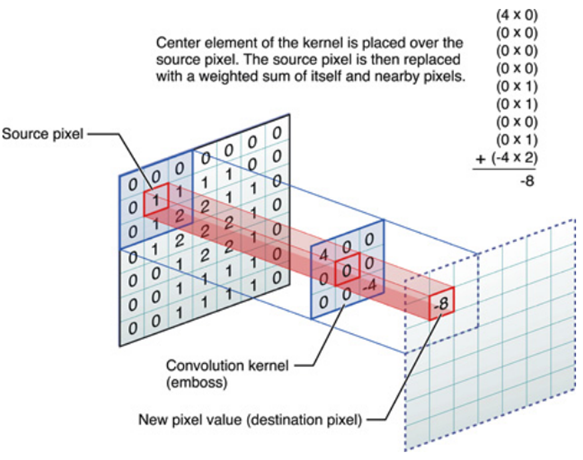对图像和滤波矩阵进行逐个元素相乘再求和的操作就相当于将一个二维的函数移动到另一个二维函数的所有位置，这个操作就叫卷积或者协相关。卷积和协相关的差别是，卷积需要先对滤波矩阵进行180的翻转，但如果矩阵是对称的，那么两者就没有什么差别了。
Correlation 和 Convolution可以说是图像处理最基本的操作，但却非常有用。这两个操作有两个非常关键的特点：它们是线性的，而且具有平移不变性shift-invariant。平移不变性指我们在图像的每个位置都执行相同的操作。线性指这个操作是线性的，也就是我们用每个像素的邻域的线性组合来代替这个像素。这两个属性使得这个操作非常简单，因为线性操作是最简单的，然后在所有地方都做同样的操作就更简单了。
实际上，在信号处理领域，卷积有广泛的意义，而且有其严格的数学定义，但在这里不关注这个。
2D卷积需要4个嵌套循环4-double loop，所以它并不快，除非我们使用很小的卷积核。这里一般使用3x3或者5x5。而且，对于滤波器，也有一定的规则要求：
1）滤波器的大小应该是奇数，这样它才有一个中心，例如3x3，5x5或者7x7。有中心了，也有了半径的称呼，例如5x5大小的核的半径就是2。
2）滤波器矩阵所有的元素之和应该要等于1，这是为了保证滤波前后图像的亮度保持不变。当然了，这不是硬性要求了。
3）如果滤波器矩阵所有元素之和大于1，那么滤波后的图像就会比原图像更亮，反之，如果小于1，那么得到的图像就会变暗。如果和为0，图像不会变黑，但也会非常暗。
4）对于滤波后的结构，可能会出现负数或者大于255的数值。对这种情况，我们将他们直接截断到0和255之间即可。对于负数，也可以取绝对值。
神奇的卷积核
上面说到，对图像的滤波处理就是对图像应用一个小小的卷积核，那这个小小的卷积核到底有哪些魔法。下面我们一起来领略下一些简单但不简单的卷积核的魔法。

1、啥也不做

哈哈，大家可以看到啥了吗？这个滤波器啥也没有做，得到的图像和原图是一样的。因为只有中心点的值是1。邻域点的权值都是0，对滤波后的取值没有任何影响。下面我们动点真格的。

2、图像锐化滤波器Sharpness Filter

图像的锐化和边缘检测很像，首先找到边缘，然后把边缘加到原来的图像上面，这样就强化了图像的边缘，使图像看起来更加锐利了。这两者操作统一起来就是锐化滤波器了，也就是在边缘检测滤波器的基础上，再在中心的位置加1，这样滤波后的图像就会和原始的图像具有同样的亮度了，但是会更加锐利。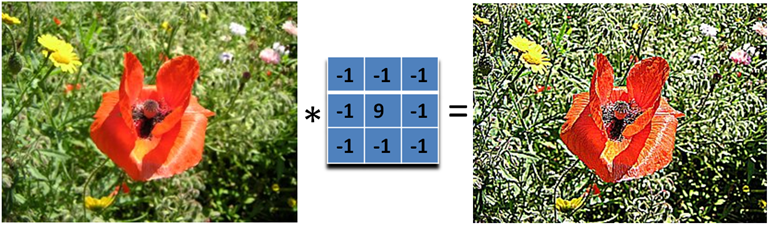我们把核加大，就可以得到更加精细的锐化效果另外，下面的滤波器会更强调边缘：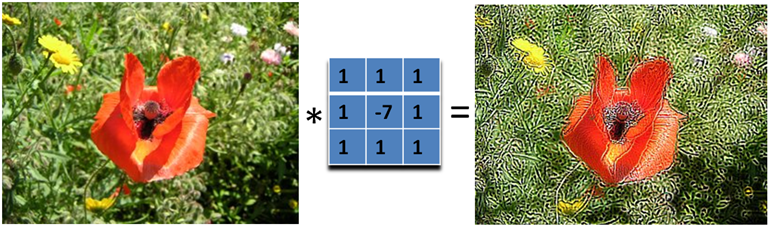主要是强调图像的细节。最简单的3x3的锐化滤波器如下：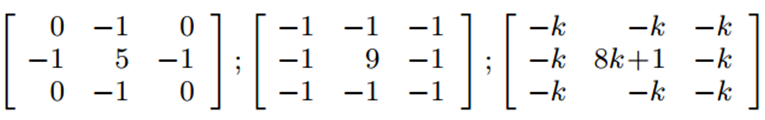大家应该也看出来了，锐化滤波器实际上就是计算当前点和周围点的差别，然后将这个差别加到原来的位置上。

3、边缘检测Edge Detection

我们要找水平的边缘：需要注意的是，这里矩阵的元素和是0，所以滤波后的图像会很暗，只有边缘的地方是有亮度的。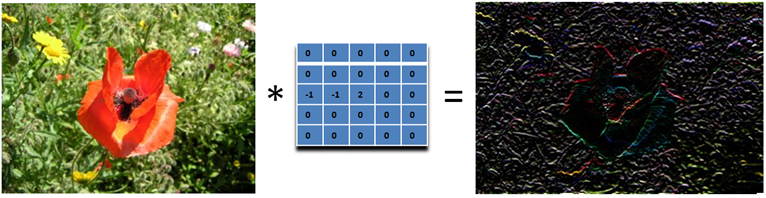为什么这个滤波器可以寻找到水平边缘呢？因为用这个滤波器卷积相当于求导的离散版本：你将当前的像素值减去前一个像素值，这样你就可以得到这个函数在这两个位置的差别或者斜率。下面的滤波器可以找到垂直方向的边缘，这里像素上和下的像素值都使用：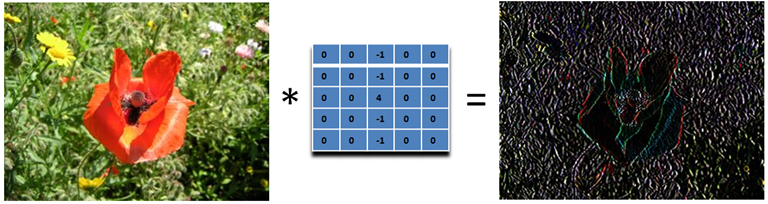再下面这个滤波器可以找到45度的边缘：取-2不为了什么，只是为了让矩阵的元素和为0而已。那下面这个滤波器就可以检测所有方向的边缘: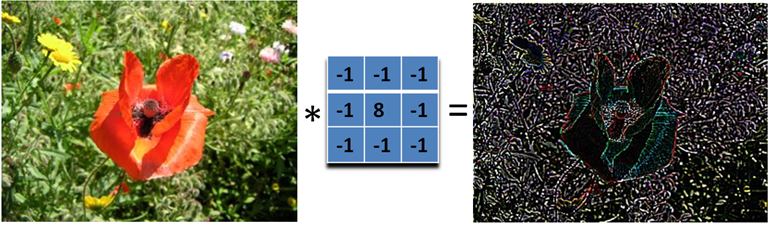为了检测边缘，我们需要在图像对应的方向计算梯度。用下面的卷积核来卷积图像，就可以了。但在实际中，这种简单的方法会把噪声也放大了。另外，需要注意的是，矩阵所有的值加起来要是0.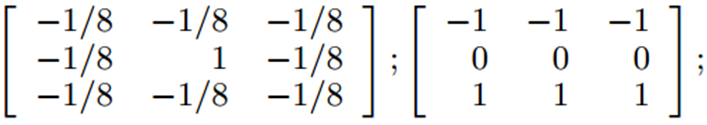4、浮雕Embossing Filter

浮雕滤波器可以给图像一种3D阴影的效果。只要将中心一边的像素减去另一边的像素就可以了。这时候，像素值有可能是负数，我们将负数当成阴影，将正数当成光，然后我们对结果图像加上128的偏移。这时候，图像大部分就变成灰色了。

下面是45度的浮雕滤波器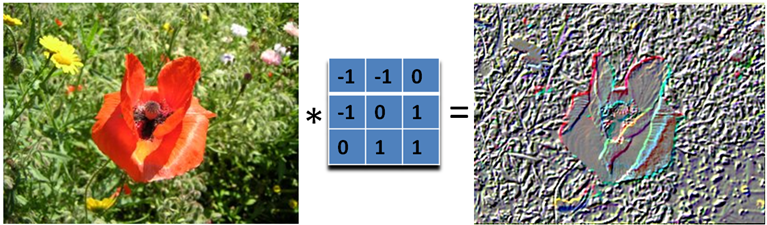我们只要加大滤波器，就可以得到更加夸张的效果了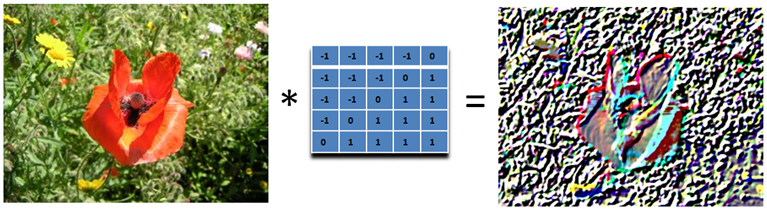这种效果非常的漂亮，就像是将一副图像雕刻在一块石头上面一样，然后从一个方向照亮它。它和前面的滤波器不同，它是非对称的。另外，它会产生负数值，所以我们需要将结果偏移，以得到图像灰度的范围。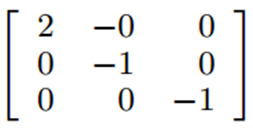A：原图像。B：锐化。C：边缘检测。D：浮雕

5、运动模糊Motion Blur
运动模糊可以通过只在一个方向模糊达到，例如下面9x9的运动模糊滤波器。注意，求和结果要除以9。这个效果就好像，摄像机是从左上角移动的右下角。

看了一些好玩的滤波器后我们可以进入主题了，首先来看均值模糊：
均值模糊Box Filter (Averaging)

我们可以将当前像素和它的四邻域的像素一起取平均，然后再除以5，或者直接在滤波器的5个地方取0.2的值即可，如下图：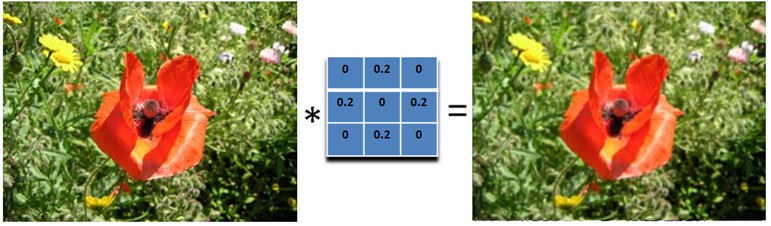可以看到，这个模糊还是比较温柔的，我们可以把滤波器变大，这样就会变得粗暴了：注意要将和再除以13.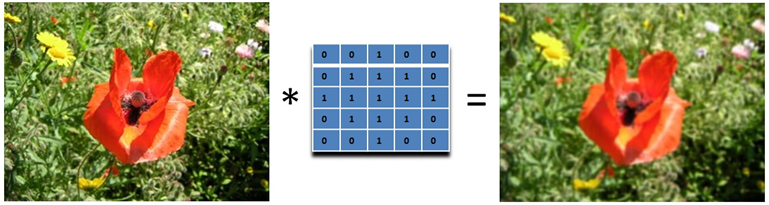所以，如果你想要更模糊的效果，加大滤波器的大小即可。或者对图像应用多次模糊也可以。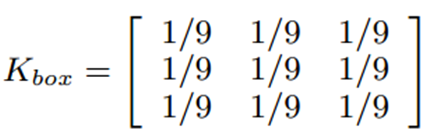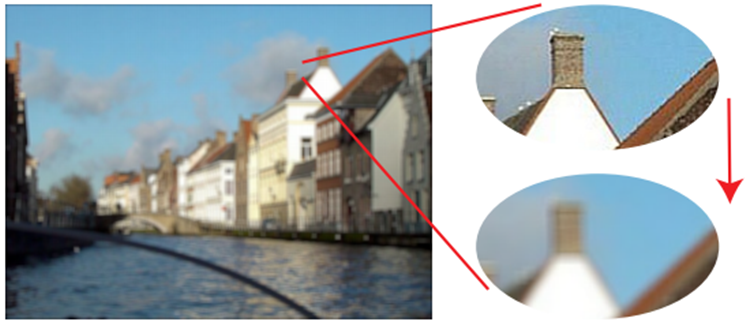高斯模糊
其实模糊滤波器就是对周围像素进行加权平均处理，均值模糊很简单，周围像素的权值都相同，所以不是很平滑。高斯模糊就有这个优点，所以被广泛用在图像降噪上。特别是在边缘检测之前，都会用来移除细节。那么下面我们就看看高斯模糊的权值是如何分配的。

正态分布的权重正态分布显然是一种可取的权重分配模式。在图形上，正态分布是一种钟形曲线，越接近中心，取值越大，越远离中心，取值越小。计算平均值的时候，我们只需要将"中心点"作为原点，其他点按照其在正态曲线上的位置，分配权重，就可以得到一个加权平均值。
高斯函数上面的正态分布是一维的，图像都是二维的，所以我们需要二维的正态分布。
正态分布的密度函数叫做"高斯函数"（Gaussian function）。它的一维形式是：其中，μ是x的均值，σ是x的标准差。因为计算平均值的时候，中心点就是原点，所以μ等于0。即：根据一维高斯函数，可以推导得到二维高斯函数：有了这个函数 ，就可以计算每个点的权重了。
假定中心点的坐标是（0,0），那么距离它最近的8个点的坐标如下：更远的点以此类推。下面就是5*5的高斯滤波器和平滑效果：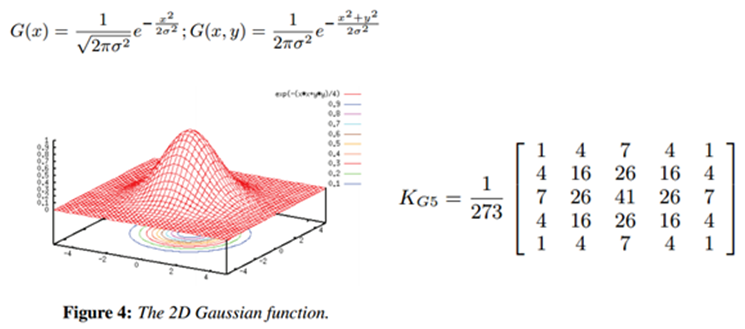展开全文• 一些基本数字图像处理算法 所有的图像算法都在DIPAlgorithm类中，并且所有算法都为抽象成员函数。我已经按照java注释规范为所有方法添加使用说明注释，具体实现可见于DIPAlgorithm.java，这里只做算法说明。 1 图像...
一些基本数字图像处理算法
版权声明：本文为原创文章，未经博主允许不得用于商业用途。
所有的图像算法都在DIPAlgorithm类中，并且所有算法都为抽象成员函数。我已经按照java注释规范为所有方法添加使用说明注释，具体实现可见于DIPAlgorithm.java，这里只做算法说明。
1 图像扭曲模仿PS的扭曲功能，通过建立一个三角形映射网格实现对图像的扭曲。
如上图，一共设置了45个控制点围成74个三角形网格
扭曲即形变处理其实是寻找一个函数，以所有网格顶点原始坐标为输入，扭曲后所有网格顶点坐标为输出。为了简化计算任务，采用控制栅格插值方法，对每个三角网格独立计算映射关系，如下图：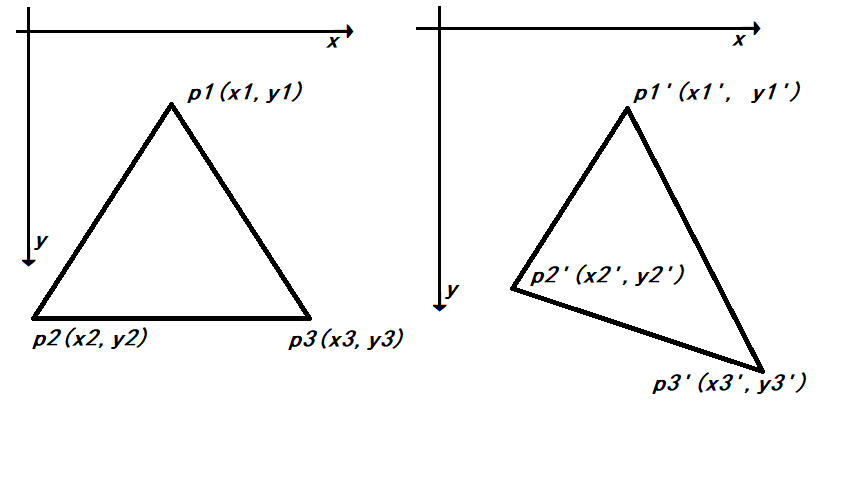即求解矩阵$M$满足$MA = B$，其中$A$为原顶点的齐次矩阵：
$A = \begin{bmatrix} x_{1} & y_{1} & 1 \\ x_{2} & y_{2} & 1 \\ x_{3} & y_{3} & 1 \\ \end{bmatrix}$
B为形变后顶点的其次矩阵：
$B = \lbrack\begin{matrix} x_{1}^{'} & x_{2}^{'} & x_{3}^{'} \\ y_{1}^{'} & y_{2}^{'} & y_{3}^{'} \\ \end{matrix}\rbrack$
M即为$2 \times 3$的映射矩阵，且由于三角形三点不共线，因此A为可逆阵，
$M = BA^{- 1}$
对于三角形中的点$p\left( x,\ y \right)$，其映射后坐标$p^{'} = M\begin{bmatrix} x \\ y \\ 1 \\ \end{bmatrix}$
2 直方图计算
直方图计算实际上即求图像的概率密度函数PDF，只需遍历一次所有像素点即可获得。
3 直方图均衡化算法
对于连续图像直方图均衡化其实是种点运算f,
对不同灰度值做映射，使得所有像素频率相等。
对于点运算f，有如下性质：
$D_{B} = f\left( D_{A} \right),\ H_{B}\left( D_{B} \right)\Delta D_{B} = H_{A}\left( D_{A} \right)\Delta D_{A}$
其中D为灰度值，H即为灰度值在图像中的频数，整理可得
$H_{B}\left( D_{B} \right) = \frac{H_{A}\left( D_{A} \right)\Delta D_{A}}{\Delta D_{B}} = \frac{H_{A}\left( D_{A} \right)}{\frac{\Delta D_{B}}{\Delta D_{A}}} = \frac{H_{A}\left( D_{A} \right)}{\frac{dD_{B}}{dD_{A}}}$
$= \frac{H_{A}\left( D_{A} \right)}{f'(D_{A})} = \frac{H_{A}\left( f^{- 1}\left( D_{B} \right) \right)}{f'(f^{- 1}(D_{B}))}$
即：
寻找函数f使得$H_{B}(D)$为常数$\frac{A_{0}}{D_{m}},A_{0},D_{m}$。
由(1)可知，KaTeX parse error: Undefined control sequence: \
at position 58: …\right)}{f'(D)}\̲
̲\Rightarrow f^{…
即$f\left( D \right) = D_{m}CDF(D)$，CDF即累积分布函数
因此只需求得直方图的前序和即可获得映射关系。
4 图像灰度化
目前比较符合人眼的灰度化权重为0.299、0.578和0.114，为了加速计算使用近似公式$D = (3r + g + 6b)/10$
5 图像二值化
我使用的二值化算法为OSTU大律二值化算法。二值化操作即利用分割阈值u，将图片分为前景后景两部分。OSTU大律法认为使得前景像素和背景像素灰度方差g最大的阈值即为最佳分割阈值。
$g = w_{0}w_{1}\left( u_{0} - u_{1} \right)^{2}$
其中$w_{0},\ w_{1}$为前景、后景在图像中的比例，KaTeX parse error: Undefined control sequence: \
at position 7: u_{0},\̲
̲u_{1}为前景、后景的平均灰度。
在实现时，只需遍历所有灰度，利用CDF求出每种灰度的方差，取最大者作为阈值即可。
6 前景分离
目前主流的前景分离为深度学习算法。这里只使用了最基本的阈值分离法，分别为RGB三个通道设置不同阈值，将小于阈值的像素作为背景，大于阈值的作为前景。
7 滤波
我使用的滤波方法是高斯滤波和中值滤波，高斯滤波即使用二维高斯函数作为滤波函数，中值滤波即使用邻域的中位数作为滤波函数。
高斯滤波器为线性滤波器，可以有效消除高斯噪声。由于高斯函数离中值越近权重越大，因此相对于均值滤波器更加柔和，对边缘的保留效果更好。这里我使用的是如下矩阵做卷积：
$\begin{bmatrix} 1 & 2 & 3 & 2 & 1 \\ 2 & 4 & 6 & 4 & 2 \\ 3 & 6 & 7 & 6 & 3 \\ 2 & 4 & 6 & 4 & 2 \\ 1 & 2 & 3 & 2 & 1 \\ \end{bmatrix}$
中值滤波器为非线性滤波器，可以有效的去除椒盐噪声和斑点噪声并且不会使图像变模糊。
8 形态学扩张和腐蚀
形态学腐蚀可记为$\text{AΘB}$，其中A为输入图像，B为结构单元。对于二值图像，当且仅当当前像素点满足腐结构单元时才会被保留。对于灰度图像，则可类比为最小值，即
$f\Theta b\left( x,y \right) = min\{ f\left( x - x^{'},\ y - y^{'} \right) - b(x^{'},y')|(x^{'},y^{'} \in D_{b})\}$
形态学扩张可看作腐蚀的逆操作,记作$A\bigoplus B$，对于二值图像，将每个有效像素点的邻域结构单元置1，对于灰度图像则取最大值，即
$f\bigoplus b\left( x,y \right) = max\{ f\left( x - x^{'},\ y - y^{'} \right) - b(x^{'},y')|(x^{'},y^{'} \in D_{b})\}$
本程序将结构单元b统一设定为5*5矩形。
通过扩张和腐蚀的结合可实现结构开运算（$AoB = \left( \text{AΘB} \right)\bigoplus B$）和结构闭运算（$AoB = \left( A\bigoplus B \right)\text{ΘB}$）对图像进行粗化、细化、滤波等处理
9 傅里叶变换和滤波
变换公式
傅里叶变换可以将信号从时域转换到频域，因此可以看出许多时域中不明显的特征。二维傅里叶变换（CFT）公式如下：
$F\left( u,v \right) = \iint_{}^{}{f\left( x,y \right)e^{- 2\pi\overrightarrow{j}(ux + vy)}}\text{dxdy}$
其中${\overrightarrow{j}}^{2} = - 1,f,F$，同样二维傅里叶逆变换公式如下:
$f\left( x,y \right) = \iint_{}^{}{F\left( u,v \right)e^{2\pi\overrightarrow{j}(ux + vy)}}\text{dudv}$
对于离散函数，可以定义离散二维傅里叶变换（DFT）和逆变换：
$G\left( m,n \right) = \frac{1}{\sqrt{\text{MN}}}\sum_{\begin{matrix} 0 \leq \ i\ \leq \ M - 1 \\ 0 < k < N - 1\ \\ \end{matrix}}^{}{g\left( i,k \right)e^{- 2\pi\overrightarrow{j}(\frac{\text{im}}{M} + \frac{\text{jn}}{N})}}$
$g\left( i,k \right) = \frac{1}{\sqrt{\text{MN}}}\sum_{\begin{matrix} 0 \leq \ m\ \leq \ M - 1 \\ 0 < n < N - 1\ \\ \end{matrix}}^{}{g\left( m,n \right)e^{2\pi\overrightarrow{j}(\frac{\text{im}}{M} + \frac{\text{jn}}{N})}}$
DFT可以理解为对连续二维信号进行了频率为M,
N的采样，之后通过计算其和频域空间M*N个基向量的相关性（在该方向投影）将时域信号映射到频域。iDFT可以理解为通过M*N个基向量合成原始时域信号。
矩阵表示
傅里叶变换实际上是一种线性变换，因此在实际计算中常常将$g$扩充为$N*N$方阵，此时DFT可以通过矩阵表示：$G = \mathcal{W}^{- 1}g\mathcal{W},\mathcal{W}_{\text{ik}} = \frac{1}{N}e^{2\pi\overrightarrow{j}\frac{\text{ik}}{N}}$。
易知$\mathcal{W}_{\text{ik}} = \mathcal{W}_{\text{ki}}$，且为正交矩阵，因此$\mathcal{W}$为酉矩阵，即$\mathcal{W}^{- 1} = \left( \mathcal{W}^{*} \right)^{T} = \mathcal{W}^{*}$，$G = \mathcal{W}^{*}g\mathcal{W}$，其中$F^{*}F$。
由于傅里叶变换为酉变换，即$\mathcal{W}^{t} = \mathcal{W}^{- 1}$
图像的傅里叶变换
对于二维图片可以看作二维矩阵，因此可以进行DFT。二维图片经过DFT后获得的复矩阵的模矩阵可以表示每个频率信号的强度（也可看作先做自相关后再进行傅里叶变换），经过适当处理即可转化为灰度能量谱图片。
线性噪声在频域中通常为点或线，因此可以通过傅里叶变换后进行滤波再通过逆变换复原图片。
算法实现
在实际实现时，根据欧拉公式，$e^{- \overrightarrow{j}t} = cost - \overrightarrow{j}\text{sint},\ e^{\overrightarrow{j}t} = cost + \overrightarrow{j}\text{sint}$，因此傅里叶变换的核矩阵可以表示为$\mathcal{W}_{\text{ik}} = \frac{\cos\left( 2\pi ik \right) - \overrightarrow{j}\sin\left( 2\pi ik \right)}{N}$，为方便运算将$\mathcal{W}$分解为虚部系数$\mathcal{W}_{\text{lm}}$和实部系数$\mathcal{W}_{\text{re}}$，其中则$\mathcal{W} = \mathcal{W}_{\text{re}} + \overrightarrow{j}\mathcal{W}_{\text{lm}}$。变换结果同样分解为$G = G_{\text{re}} + \overrightarrow{j}G_{\text{lm}}$，则DFT可以表示为:
$G = \mathcal{W}^{*}g\mathcal{W =}\left( \mathcal{W}_{\text{re}} - \overrightarrow{j}\mathcal{W}_{\text{lm}} \right)g\left( \mathcal{W}_{\text{re}} + \overrightarrow{j}\mathcal{W}_{\text{lm}} \right) = \mathcal{W}_{\text{re}}g\mathcal{W}_{\text{re}} + \mathcal{W}_{\text{lm}}g\mathcal{W}_{\text{lm}} - \overrightarrow{j}\left( \mathcal{W}_{\text{lm}}g\mathcal{W}_{\text{re}} + \mathcal{W}_{\text{re}}g\mathcal{W}_{\text{lm}} \right)$
$\left\{ \begin{matrix} G_{\text{re}} = \mathcal{W}_{\text{re}}g\mathcal{W}_{\text{re}} + \mathcal{W}_{\text{lm}}g\mathcal{W}_{\text{lm}} \\ G_{\text{lm}} = - \mathcal{W}_{\text{lm}}g\mathcal{W}_{\text{re}} - \mathcal{W}_{\text{re}}g\mathcal{W}_{\text{lm}} \\ \end{matrix} \right.\$
同理，iDFT可以表示为：
$g = \left( \mathcal{W}_{\text{re}} + \overrightarrow{j}\mathcal{W}_{\text{lm}} \right)(G_{\text{re}} + {\overrightarrow{j}G}_{\text{lm}})\left( \mathcal{W}_{\text{re}} - \overrightarrow{j}\mathcal{W}_{\text{lm}} \right)$
其中，为了将能量谱转化为可见的灰度图，为能量谱取对数值进行归一化。且由于在频域中两个维度频率都为0时（即$\mathcal{W}_{00}$处）为图像能量的总和，因此通过$log(e + 1)*\frac{256}{\log\left( \mathcal{W}_{00} + 1 \right)}$可以做进一步归一化。
算法代码可见github


展开全文数字图像处理
• 也称光学图像处理，它是利用光学透镜或光学照相方法对模拟图像进行的处理，其实时性强、速度快、 处理信息量大、分辨率高，但是处理精度低，灵活度差，难有判断功能 。 数字图像处理： 即利用计算机对数字...
一、基本概念

1.1 图像分类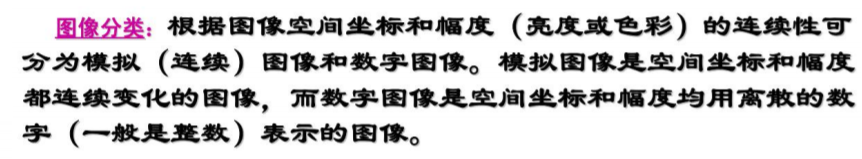1.2 图像处理方法

模拟图像处理：
也称光学图像处理，它是利用光学透镜或光学照相方法对模拟图像进行的处理，其实时性强、速度快、
处理信息量大、分辨率高，但是处理精度低，灵活度差，难有判断功能 。

数字图像处理：
即利用计算机对数字图像进行处理 ，它具有精度高、处理内容丰富、方法易变、灵活度高等优点。
但是它的处理速度受到计算机和数字器件的限制，一般也是串行处理，因此处理速度较慢。

光电结合处理：
用光学方法完成运算量巨大的处理（如频谱变换等），而用计算机对光学处理结果（如频谱）进行分析判断等处理。
该方法是前两种方法的有机结合，它集结了二者的优点。光电结合处理是。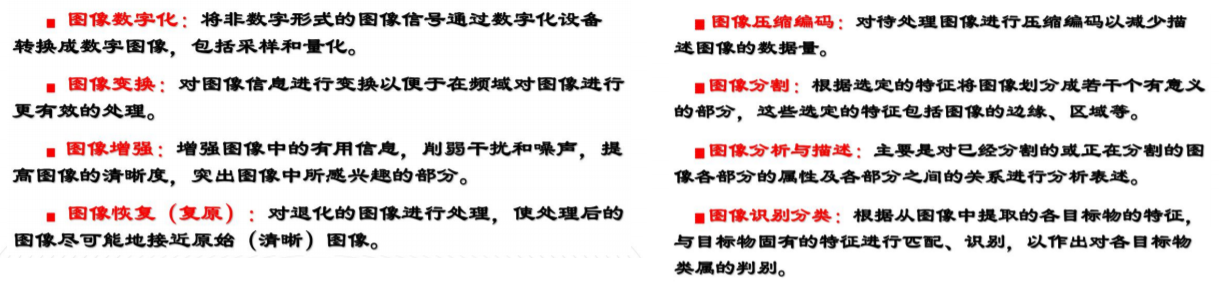1.3 颜色表示方法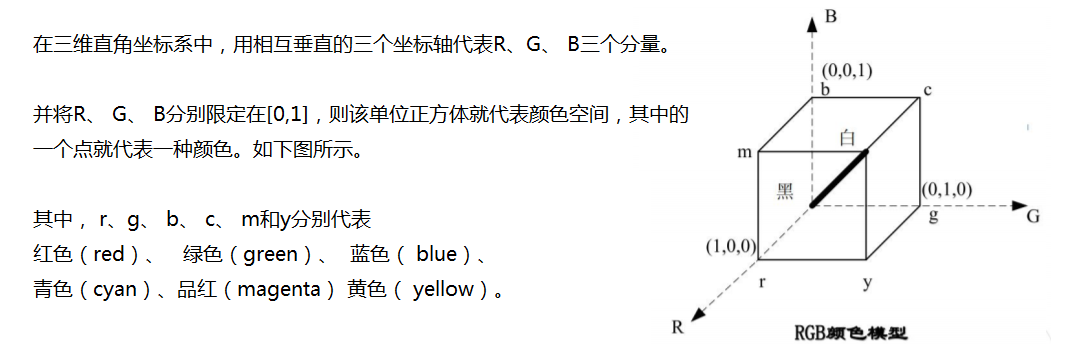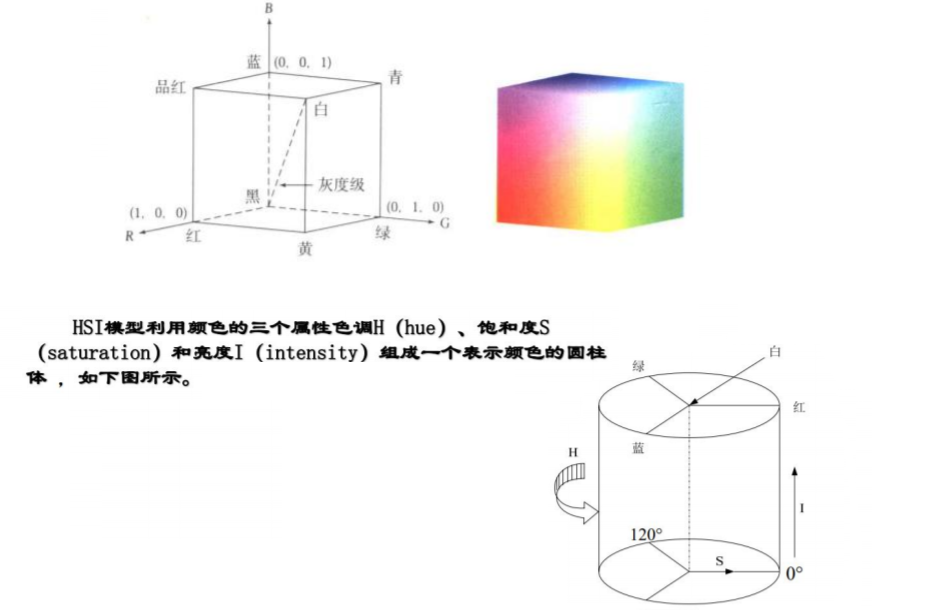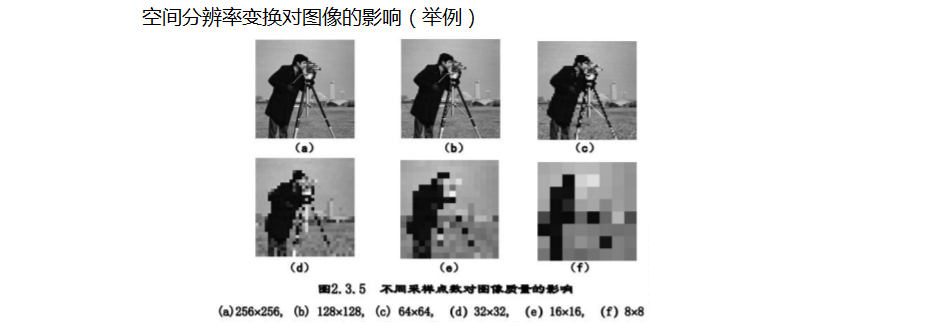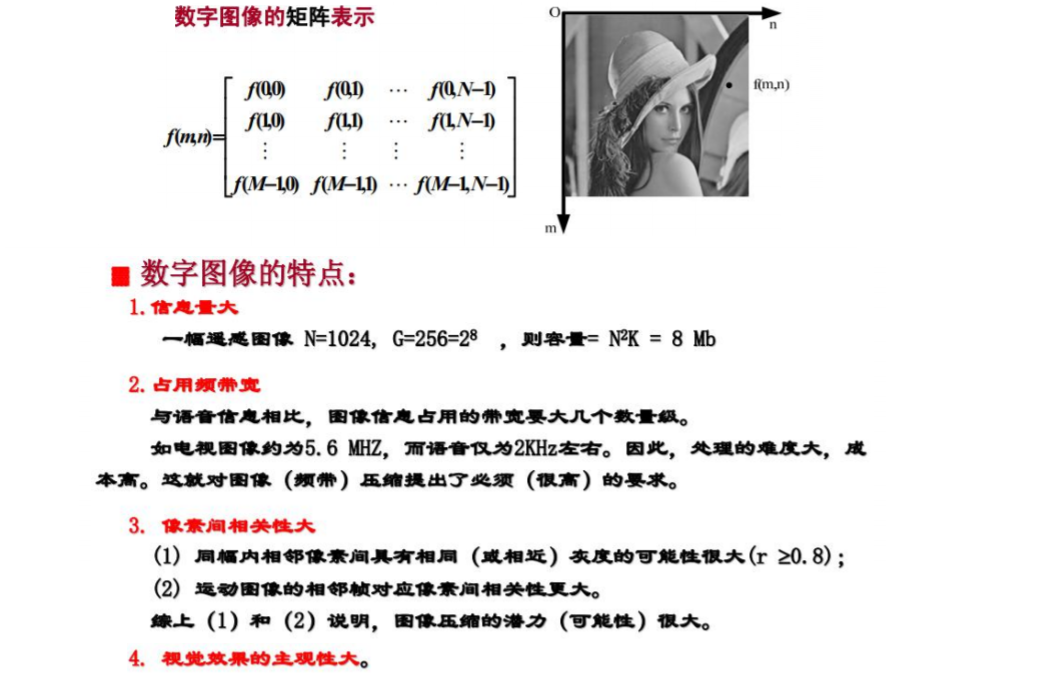1.4 图像的几何变换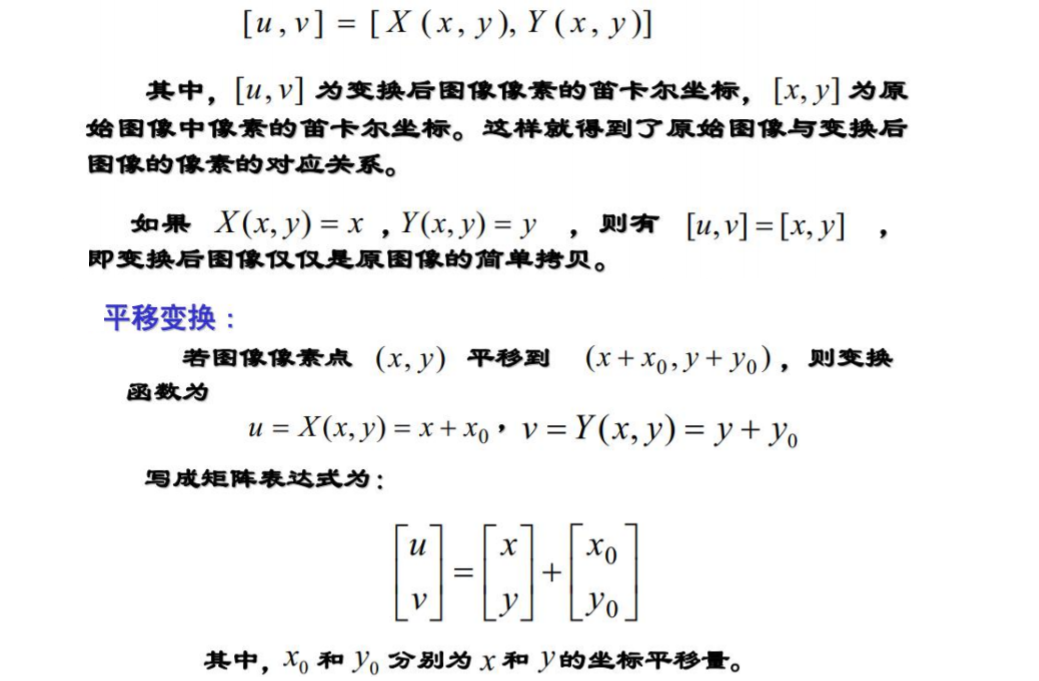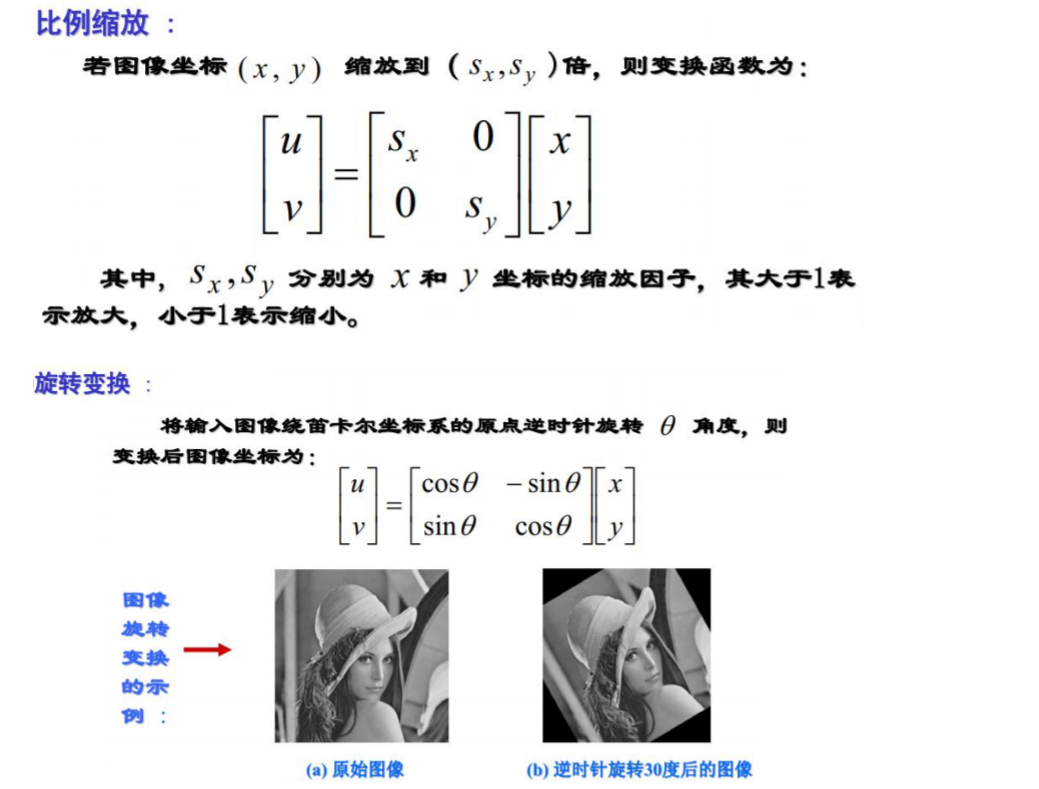仿射变换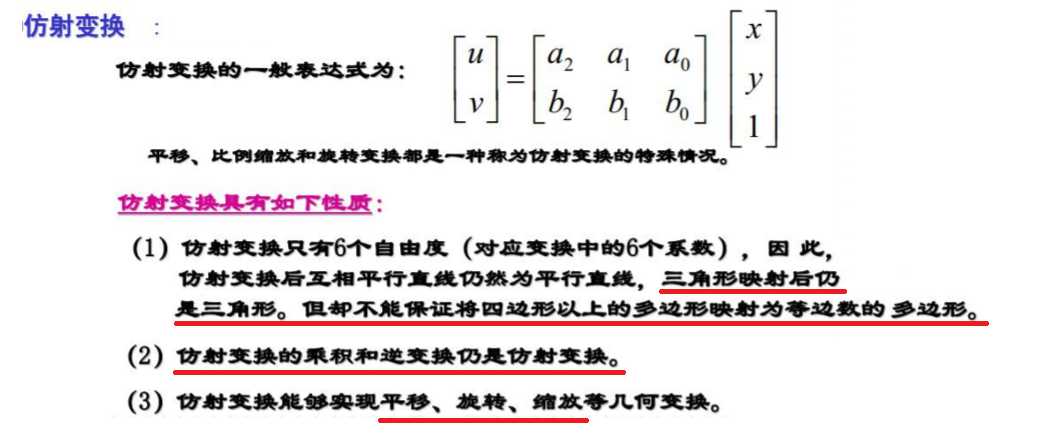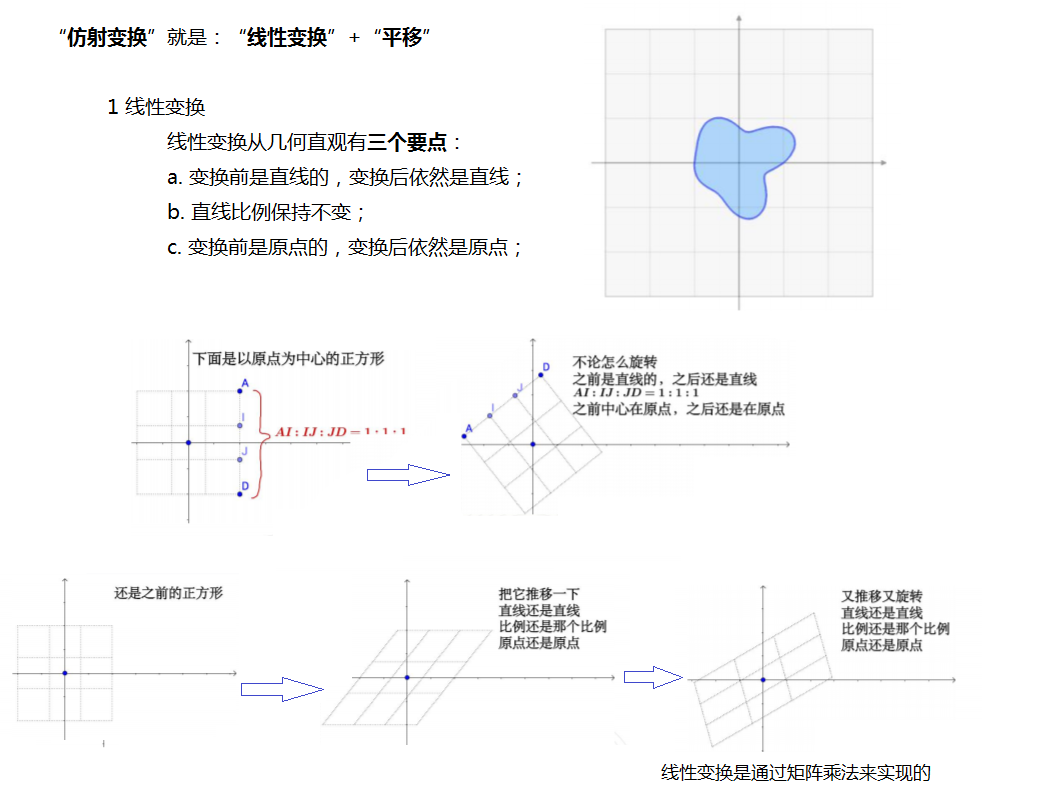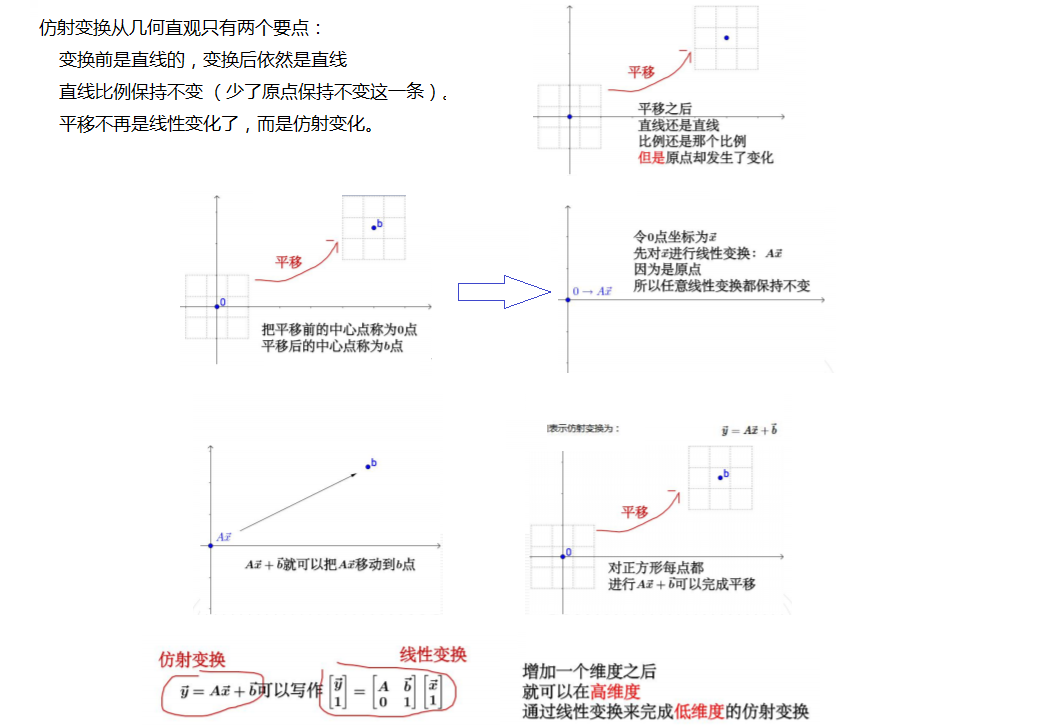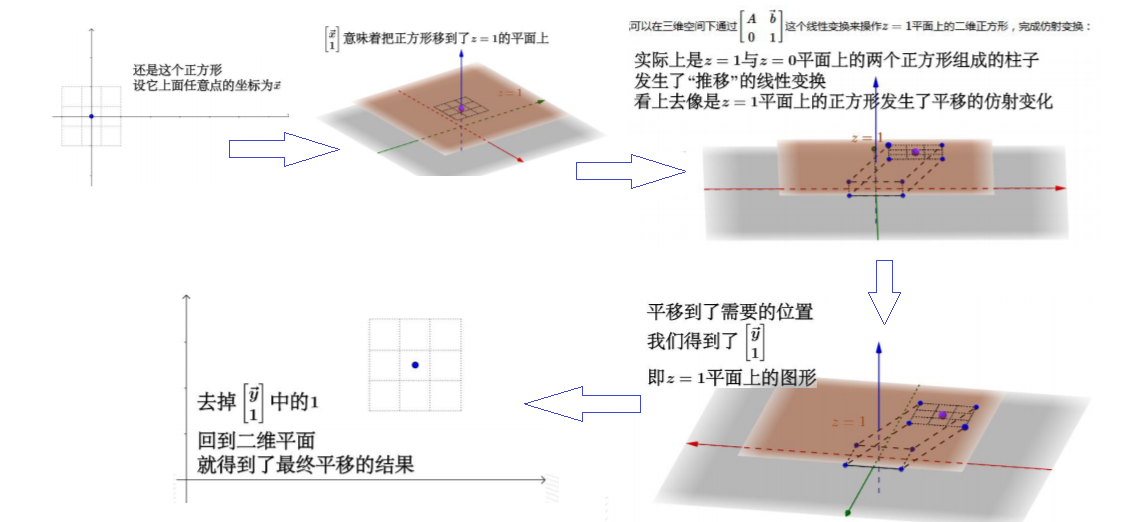展开全文图像处理基础
• 一、学习心得： 在我学习基本滤波算法原理的时候，因为刚接触不是很理解算法具体是怎样实现的，不过在学习了图像形态学之后，发现滤波算法其实很简单。所以在此建议初学者在...而在图像处理中的滤波算法中，处理的图像处理 滤波 图像增强
• 整理了一下网上各个公司图像处理算法工程师的招聘要求：  图像处理算法工程师 职位要求 编程技能： 1、 具有较强的编程能力和良好的编程习惯， 精通c/c++编程，并熟练使用VS 或matlab开发环境； 2、 在计算机...
• 将颜色的RGB设置为相同的值即可使得图片为灰色，一般处理方法有： 1、取三种颜色的平均值 2、取三种颜色的最大值（最小值） 3、加权平均值：0.3R + 0.59G + 0.11*B for(var i = 0; i < data.length; i+=4) { var...图像处理算法
• 图像处理之霍夫变换（直线检测算法） 霍夫变换是图像变换中的经典手段之一，主要用来从图像中分离出具有某种相同特征的几何 形状（如，直线，圆等）。霍夫变换寻找直线与圆的方法相比与其它方法可以更好的减少噪 声...图像处理 算法 image float equals
• Scriptol列出了几种神奇的图像处理算法，让我们一起来看一下。 一、像素图生成向量图的算法 数字时代早期的图片，分辨率很低。尤其是一些电子游戏的图片，放大后就是一个个像素方块。Depixelizing算法可以让低...图像处理算法
• 下面简要分析了 FPGA 技术，包括 FPGA 技术原理和技术特点等，随后介绍一下FPGA 的图像处理系统算法的实现，包括存储模块、运算单元、控制模块以及数据传输模块等内容。 智能机器人、多媒体已经计算机的诞生都离不...图像处理
• 有可能有边缘的地方并非边界，也有可能边界的地方并无边缘，因为现实世界中的物体是三维的，而图像只具有二维信息，从三维二维的投影成像不可避免的会丢失一部分信息；另外，成像过程中的光照和噪声也是不可避免的...图像处理
• 主要把图像处理的一部分经典基础算法梳理并实现了一遍，主要是用VS2013+OpenCV3.1.0做的。 主要目的：一是为了把基础巩固一下，以便更高效地看论文；二是为了工作基础。 因为以前在学习和研究的过程中发现自己基础...
• 数字图像处理中常用图像分割算法有哪些？ 1.多数的图像分割算法 2.图像边缘分割 3.图像阈值分割 4.基于区域的分割 5.形态学分水岭算法 多数的图像分割算法 均是基于灰度值的不连续和相似的性质。在前者中，...
• 本文索引： 一 什么是卷积 二 相关算子 三 卷积算子 ... 卷积核（算子）是用来做图像处理时的矩阵,图像处理时也称为掩膜，是与原图像做运算的参数。卷积核通常是一个四方形的网格结构（例如3*3的矩阵或像图像处理 算法
• 图像算法开发跟普通程序的软件算法开发又不一样，图像算法主要是关注如何对图像进行处理，也就是关注的优化的对象不一样。图像算法开发过程，一般是： 1）在PC端进行算法验证，达到所要实现的功能。 2）算法移植...图像处理
• 误差扩撒法在数据处理中经常碰到，尤其是用于图像处理中，降低色彩的深度，这个算法还是比较有用的。
• &nbsp;&nbsp;&nbsp;&nbsp;&nbsp;&nbsp;&nbsp;&nbsp;&nbsp;&nbsp;&nbsp;&nbsp;&nbsp;&nbsp;&nbsp;&nbsp;&nbsp;&nbsp;...&a
• 作者:July 二零一一年二月二十六日。 ...参考：百度百科、维基百科、vc数字图像处理。...--------------------------------------------------...数字图像处理领域的二十四个典型算法及vc实现、第一章 一、256色转灰机器学习 图像分割